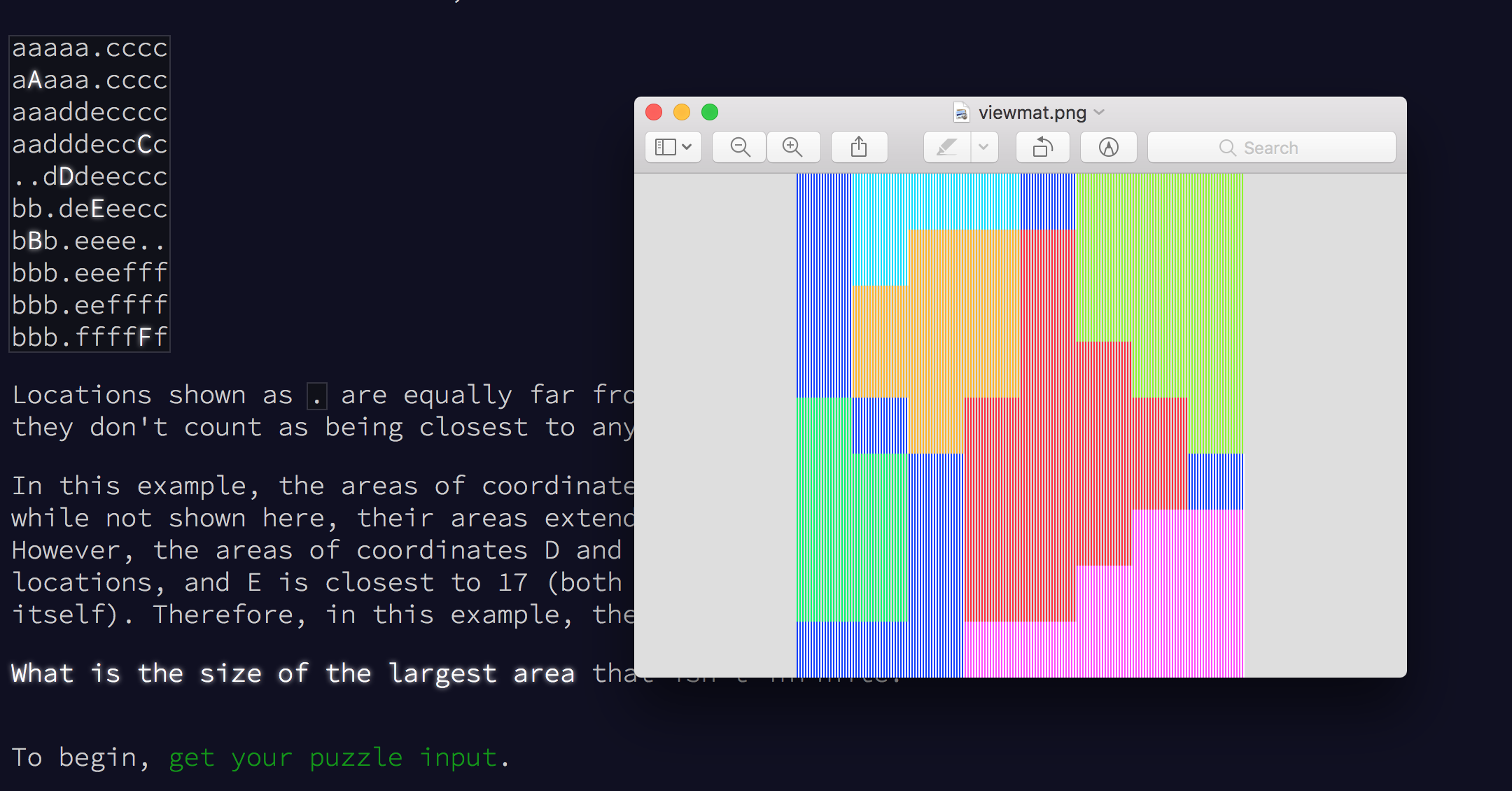Today’s Advent of Code challenge asked us to plot a Manhattan distance Voronoi diagram of a collection of points, and to find the area of the largest, but finite, cell within our diagram.

I’ll be honest. This was a difficult problem for me to solve with my current level of J-fu.

My high level plan of attack was to build up a “distance matrix” for each of the points in our diagram. The location of a point would have a value of `0`, neighbors would have a value of `1`, and so on. In theory, I’d be able to write a verb that combines two matrices and returns a new matrix, with tied distances represented as `_1`. I could insert (`/`) this verb between each of my matrices, reducing them down to a final matrix representing our Voronoi diagram.

I wrote some quick helper verbs to find the distance between two points:

``````    d =. [: +/ |@-
``````

Find the width and height of the bounding rectangle of my input:

``````    wh =. 1 + >./ - <./
``````

Generate the set of coordinates for my matrices (this one took some serious trial and error):

``````    coords =. 3 : 0
'w h' =. (1 + >./ - <./) y
(<./y) +"1  (i. h) ,"0~ ((h,w) \$ i. w)
)
``````

And to fill that matrix with the distances to a given point:

``````    grid =. 4 : 0
(x d ])"1 coords > y
)
``````

The process of adding together two matrices was more complicated. I went through many horribly broken iterations of this process, but I finally landed on this code:

``````    compare =. 4 : 0
'vx ix' =. x
'vy iy' =. y
vx = vy
)

tie =. 4 : 0
(0 {:: x);_1
)

pick =. 4 : 0
'vx ix' =. x
'vy iy' =. y
v =. vx ((y"_) ` (x"_) @. <) vy
)

x (pick ` tie @. compare) y
)
``````

With that, I could compute my final grid:

``````    numbers =. ". input
grids =. ([ ;"0 i.@#) numbers grid"1 <numbers
``````

Our `sum` keeps track of closest input point at each position on our grid, and also the actual distance value to that point. The closest input point is what we’re trying to count, so it’s probably the more interesting of the two values:

``````    groups =. (1&{::)"1 sum
0  0  0 0 _1 2 2  2
0  0  3 3  4 2 2  2
0  3  3 3  4 2 2  2
_1  3  3 3  4 4 2  2
1 _1  3 4  4 4 4  2
1  1 _1 4  4 4 4 _1
1  1 _1 4  4 4 5  5
1  1 _1 4  4 5 5  5
1  1 _1 5  5 5 5  5
``````

We could even render the grid using J’s `viewmat` utility. Awesome!Our sample inputs, visualized with viewmat.

Using `viewmat` to visualize my matricies like this actually helped my find and fix a bug in my solution incredibly quickly. I’m a big fan and plan on using it more in the future.

Because of how Manhattan distance works, cells with infinite volume are the cells that live on the border of our final matrix.

To find those infinite groups that live along the edges of my final matrix, I appended the edge of each edge of my matrix together and returned the nub of those values. I found the idea for this matrix rotation helper from this video on J I watched many months ago. I’m glad I remembered it!

``````    rot =. [: |. |:

edges =. 3 : 0
top =. 0 { y
right =. 0 { rot^:1 y
bottom =. 0 { rot^:2 y
left =. 0 { rot^:3 y
~. top , right , bottom , left
)
``````

To find my final answer, I raveled my matrix, removed the infinite groups, used the “key” (`/.`) adverb to count the size of each group, and returned the size of the largest group.

``````    without =. _1 , edges groups
raveled =. ,groups
0 0 {:: \:~ ({. ;~ #)/.~ raveled #~ -. raveled e. without
``````

This definitely isn’t the most efficient solution, but it works. At this point, I’m happy with that.

## Part Two

Part two turned out to be much easier than part one. We simply needed to iterate over each point in our grid, counting the total distance to each of our input points. The set of points that was less than a fixed number from all input points defined a circular “landing area”. We were asked to find the size of that area.

I gutted most of my part one solution and replaced the values returned by my `grid` verb with the total distance to each input point:

``````    distances =. 4 : 0
+/ ((>x) d"1~ ])"1 y
)

grid =. 3 : 0
(<y) distances"1 coords y
)
``````

Finding my final answer was as easy as calculating my grid, checking which points were less than `10000`, removing all of the `0` values, and counting the result.

``````    numbers =. ". input
# #~ , 10000 > grid numbers
``````
• Rotating a matrix (`|. |:`) is a great trick.
• `viewmat` is awesome. It very quickly helped me find and fix a bug in my solution.
• Boxes can be treated like arrays in most cases. I was under the wrong impression that a box was a single unit in terms of rank.